# sklearn.datasets.make_moons¶

sklearn.datasets.make_moons(n_samples=100, *, shuffle=True, noise=None, random_state=None)[source]

Make two interleaving half circles.

A simple toy dataset to visualize clustering and classification algorithms. Read more in the User Guide.

Parameters:
n_samplesint or tuple of shape (2,), dtype=int, default=100

If int, the total number of points generated. If two-element tuple, number of points in each of two moons.

Changed in version 0.23: Added two-element tuple.

shufflebool, default=True

Whether to shuffle the samples.

noisefloat, default=None

Standard deviation of Gaussian noise added to the data.

random_stateint, RandomState instance or None, default=None

Determines random number generation for dataset shuffling and noise. Pass an int for reproducible output across multiple function calls. See Glossary.

Returns:
Xndarray of shape (n_samples, 2)

The generated samples.

yndarray of shape (n_samples,)

The integer labels (0 or 1) for class membership of each sample.

## Examples using sklearn.datasets.make_moons¶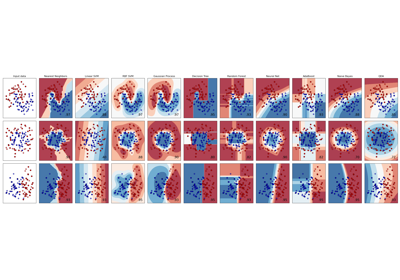Classifier comparison

Classifier comparison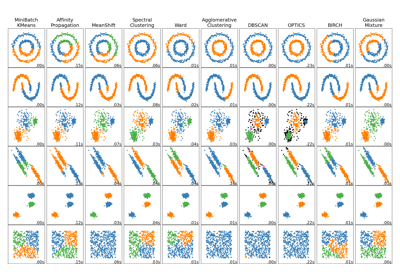Comparing different clustering algorithms on toy datasets

Comparing different clustering algorithms on toy datasets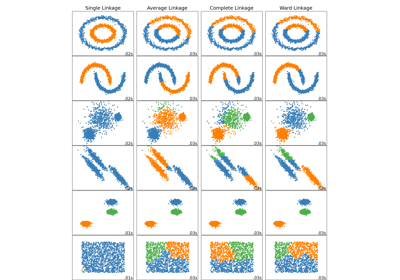Comparing different hierarchical linkage methods on toy datasets

Comparing different hierarchical linkage methods on toy datasets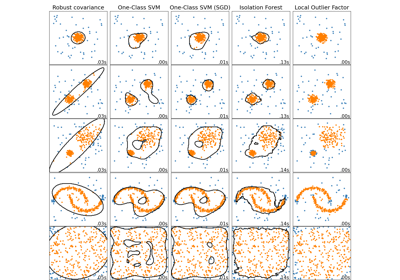Comparing anomaly detection algorithms for outlier detection on toy datasets

Comparing anomaly detection algorithms for outlier detection on toy datasets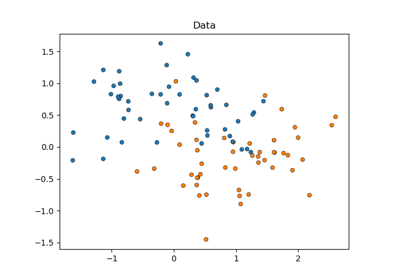Statistical comparison of models using grid search

Statistical comparison of models using grid search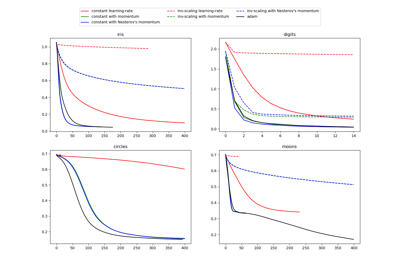Compare Stochastic learning strategies for MLPClassifier

Compare Stochastic learning strategies for MLPClassifier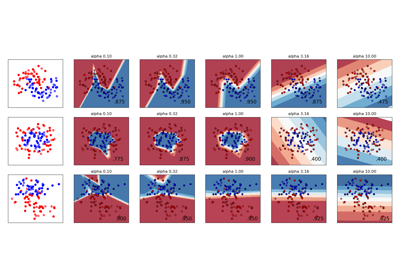Varying regularization in Multi-layer Perceptron

Varying regularization in Multi-layer Perceptron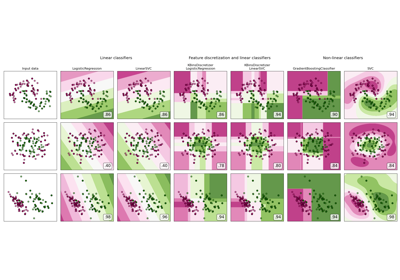Feature discretization

Feature discretization